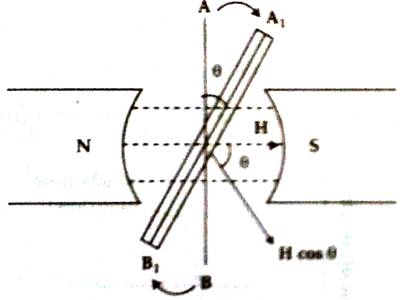Physics

# Explanation on Generation of Alternating CurrentExplanation on Generation of alternating current

There is a source of current from which the flow of current in the circuit changes direction spontaneously at a regular interval. This current is called alternating current. Alternating current describes the flow of charge that changes direction periodically. As a result, the voltage level also reverses along with the current. AC is used to deliver power to houses, office buildings, etc.

Suppose N and S are two poles of a magnet which creates a uniform magnetic field intensity of H [Figure]. Let AB be a closed loop. It is situated perpendicular to the magnetic field. The loop is rotating about its own horizontal axis with an angular velocity of ω.Suppose the loop contains n number of turns and its area is A. So, if the plane of the loop is normal to the field lines, then the magnetic flux passing through it is,

φ = nμAH

Now let us consider that the coil in time ‘t’ reaches to a position A1B2 after rotating an angle θ. Then the horizontal components of the field intensity = H cos θ.

So, magnetic flux or number of field crossing the coil,

φN = nμAH cos θ

= nμAH cos ωt

Since due to the rotation of the coil the magnetic flux passing through the coil will change, so as a result of Faraday’s electromagnetic induction electromotive force will be induced in the coil and its value is.

E = – dφN / dt

= – d/dt (nμAH cos ωt)

= nμAH ω sin ωt

= E0 sin ωt … … … (1)

Here, E0 = nµ AHω = maximum electromotive force.

Equation (1) is called a sinusoidal equation. In case of current above equation can be written as, I = I0 sin ωt. In this way’ we get alternating current.